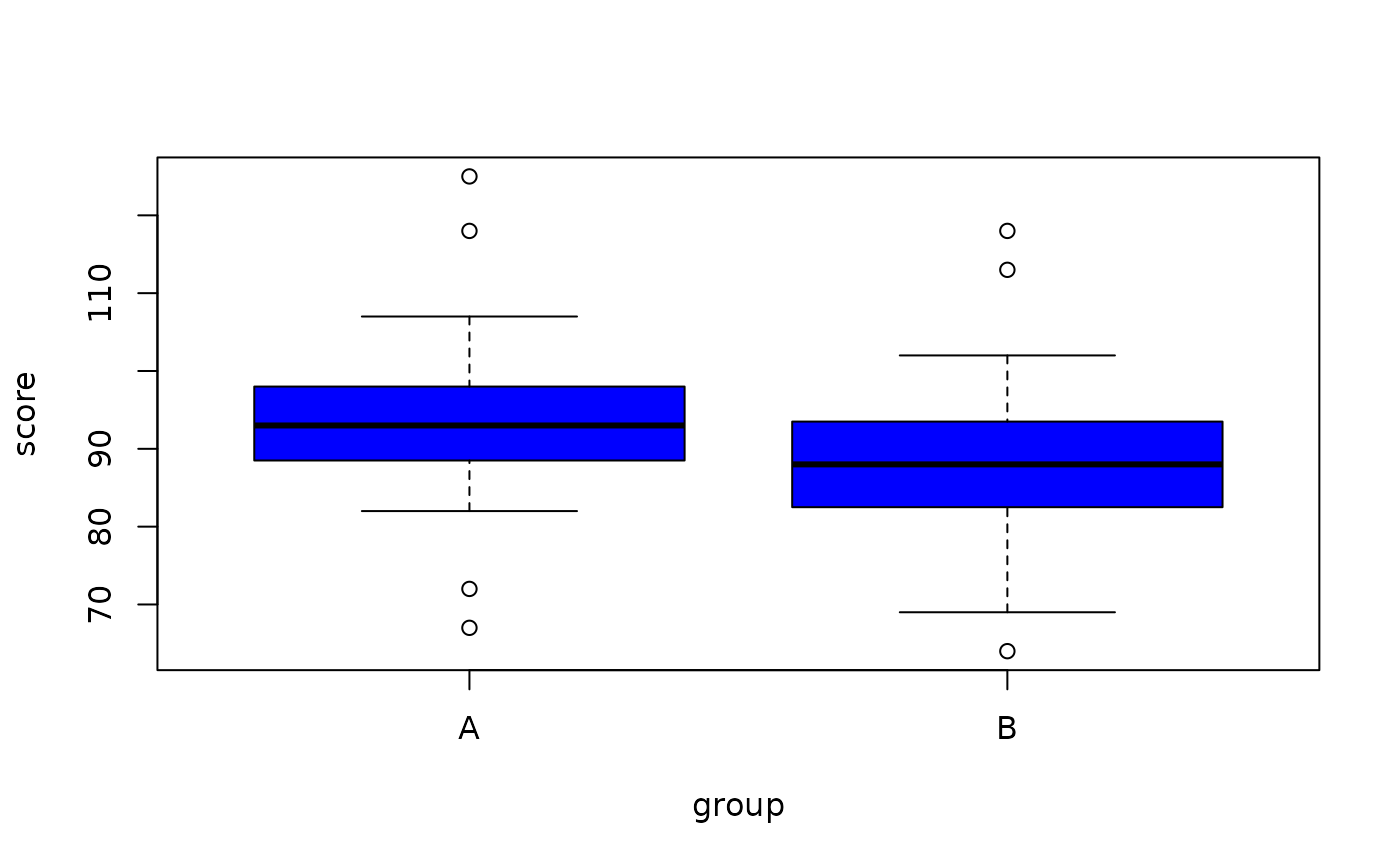Data for Exercise 7.41

Independent

## Format

A data frame/tibble with 46 observations on two variables

score

a numeric vector

group

a factor with levels A and B

## Examples


qqnorm(Independent$score[Independent$group=="A"])
qqline(Independent$score[Independent$group=="A"])qqnorm(Independent$score[Independent$group=="B"])
qqline(Independent$score[Independent$group=="B"])boxplot(score ~ group, data = Independent, col = "blue")wilcox.test(score ~ group, data = Independent)
#> Warning: cannot compute exact p-value with ties
#>
#> 	Wilcoxon rank sum test with continuity correction
#>
#> data:  score by group
#> W = 349.5, p-value = 0.06321
#> alternative hypothesis: true location shift is not equal to 0
#>